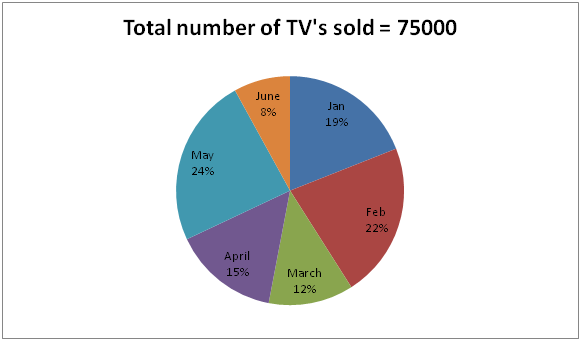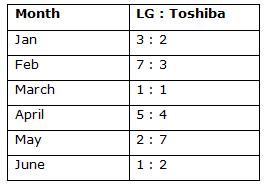# IBPS SO / NIACL AO Prelims – Quantitative Aptitude Questions Day- 59

Dear Readers, Bank Exam Race for the Year 2018 is already started, To enrich your preparation here we have providing new series of Practice Questions on Quantitative Aptitude – Section. Candidates those who are preparing for IBPS SO / NIACL AO Prelims 2018 Exams can practice these questions daily and make your preparation effective.

[WpProQuiz 4661]

1) A can finish a work in 12 days. B can finish the same work in 8 days and C in 15 days. A and B start the work but they leave after 4 days. How many days C will take to finish the remaining work?

a) 4 (7/8) days

b) 2 (1/2) days

c) 3 (5/6) days

d) 5 (¾) days

e) None of these

2) Anil purchased a computer for Rs. 35000 and sold it at a loss of 8 %, with that money he purchased another computer and sold it at the profit of 9 %. Find the overall profit/loss?

a) Rs. 114

b) Rs. 98

c) Rs. 156

d) Rs. 76

e) None of these

3) The amount obtained on Rs. 32000 at an interest 4% per annum compounded annually for certain period of time is Rs. 35995.648. Then find the time in years?

a) 3 years

b) 5 years

c) 2 years

d) 4 years

e) None of these

4) A, B and C started a business by investing an amount of Rs. 45000, Rs. 65000 and Rs. 70000 respectively. After 3 months, A invested Rs. 15000 more and B withdraw Rs. 5000. And after 4 months, C withdraws Rs. 15000. If the total profit at the end of the year is Rs. 114405, then find the share of A?

a) Rs. 35505

b) Rs. 32960

c) Rs. 28730

d) Rs. 26525

e) None of these

5) In three numbers, the first is twice the second and thrice the third. If the average of three numbers is 99, then the first number is?

a) 162

b) 134

c) 146

d) 178

e) 126

Directions (Q. 6 – 10): Study the following information carefully and answer the given questions:

The following pie chart shows the total number of TV’s sold in different months and the table shows the ratio between the total number of TV’s sold by LG and Toshiba Company.6) Total number of TV’s sold in the month of Feb and June is what percentage of total number of TV’s sold in the month of Jan, March and May together?

a) 90.90 %

b) 110.11 %

c) 54.54 %

d) 85.85 %

e) None of these

7) On which month, the highest number of LG TV’s sold?

a) Jan

b) Apr

c) May

d) Feb

e) None of these

8) Find the ratio between the total number of Toshiba TV’s sold in the month of Jan, Feb and March together to that of total number of LG TV’s sold in the month of April, May and June together?

a) 303: 245

b) 291: 236

c) 153: 202

d) 395: 478

e) None of these

9) Find the difference between the total number of TV’s sold in the month of March to that of total number of LG TV’s sold in the month of Feb and May together?

a) 5980

b) 6550

c) 4870

d) 7230

e) None of these

10) Find the total number Toshiba TV’s sold in the given six months?

a) 27890

b) 32270

c) 38150

d) 29530

e) None of these

A’s one day work = (1/12)

B’s one day work = (1/8)

C’s one day work = (1/15)

(A + B)’s one day work = (1/12) + (1/8) = 5/24

(A + B)’s 4 day work = (5/24)*4 = 5/6

Remaining work = 1/6

C can finish the remaining work in,

= > (1/6)*15 = 2 (1/2) days

C. P1 = 35000, Loss = 8 %

S.P1 = 35000 * (92/100) = 32200

With that money he purchased another computer and sold it at the profit of 9%,

C.P2 = 32200

S.P2 = 32200*(109/100) = 35098

The overall profit = 35098 – 35000 = Rs. 98

Amount = P (1 + r/100)n

35995.648 = 32000(1 + (4/100))n

35995.648/32000 = (104/100)n

35995648/32000000 = (26/25)n

8998912/8000000 = (26/25)n

(208/200)3 = (26/25)n

(26/25)3 = (26/25)n

n = 3 years

The share of A, B and C,

= > [45000*3 + 60000*9]: [65000*3 + 60000*9]: [70000*7 + 55000*5]

= > 675000: 735000: 765000

= > 45: 49: 51

Total profit = Rs. 114405

145’s = 114405

1’s = 789

The share of A = 789*45 = Rs. 35505

Let the first, second and third number be a, b and c.

First number = 2* second number

First number = 3* third number

Average of three numbers = 99

Total of three numbers = 99*3 = 297

According to the question,

a = 2b = 3c

a = 2b = > (a/b) = (2/1)

2b = 3c = > (b/c) = (3/2)

a: b: c = 6 : 3 : 2

11’s = 297

1’s = 27

First number = 6’s = 162

Direction (6-10) :

Required % = [(22 + 8) * (75000/100) / (19+12+24) (75000/100)]*100

= > (30/40)*100

= > 54.54 %

LG TV’s are,

Jan = 75000*(19/100)*(3/5) = 8550

Feb = 75000*(22/100)*(7/10) = 11550

Mar = 75000*(12/100)*(1/2) = 4500

Apr = 75000*(15/100)*(5/9) = 6250

May = 75000*(24/100)*(2/9) = 4000

June = 75000*(8/100)*(1/3) = 2000

The highest number of LG TV’s sold in the month of Feb.

The total number of Toshiba TV’s sold in the month of Jan, Feb and March together

= > 75000*(19/100)*(2/5) + 75000*(22/100)*(3/10) + 75000*(12/100)*(1/2)

= > 5700 + 4950 + 4500 = 15150

The total number of LG TV’s sold in the month of April, May and June together

= > 75000*(15/100)*(5/9) + 75000*(24/100)*(2/9) + 75000*(8/100)*(1/3)

= > 6250 + 4000 + 2000 = 12250

Required ratio = 15150: 12250 = 303: 245

The total number of TV’s sold in the month of March

= >75000 *(12/100)

= > 9000

The total number of LG TV’s sold in the month of Feb and May together

= > 75000*(22/100)*(7/10) + 75000*(24/100)*(2/9)

= > 11550 + 4000

= > 15550

Required difference = 15550 – 9000 = 6550

The total number Toshiba TV’s sold in the given six months

= > 75000*(19/100)*(2/5) + 75000*(22/100)*(3/10) + 75000*(12/100)*(1/2) + 75000*(15/100)*(4/9) + 75000*(24/100)*(7/9) + 75000*(8/100)*(2/3)

= > 5700 + 4950 + 4500 + 5000 + 14000 + 4000

= > 38150

Daily Practice Test Schedule | Good Luck

 Topic Daily Publishing Time Daily News Papers & Editorials 8.00 AM Current Affairs Quiz 9.00 AM Current Affairs Quiz (Hindi) 9.30 AM IBPS SO/NIACL AO Prelims – Reasoning 10.00 AM IBPS SO/NIACL AO Prelims – Reasoning (Hindi) 10.30 AM IBPS SO/NIACL AO Prelims – Quantitative Aptitude 11.00 AM IBPS SO/NIACL AO Prelims – Quantitative Aptitude (Hindi) 11.30 AM Vocabulary (Based on The Hindu) 12.00 PM IBPS SO/NIACL AO Prelims – English Language 1.00 PM SSC Practice Questions (Reasoning/Quantitative aptitude) 2.00 PM IBPS Clerk – GK Questions 3.00 PM SSC Practice Questions (English/General Knowledge) 4.00 PM Daily Current Affairs Updates 5.00 PM SBI PO/IBPS Clerk Mains – Reasoning 6.00 PM SBI PO/IBPS Clerk Mains – Quantitative Aptitude 7.00 PM SBI PO/IBPS Clerk Mains – English Language 8.00 PM